• 表达式中缀转后缀C++代码表达式中缀转后缀C++代码表达式中缀转后缀C++代码表达式中缀转后缀C++代码表达式中缀转后缀C++代码表达式中缀转后缀C++代码表达式中缀转后缀C++代码表达式中缀转后缀C++代码表达式中缀转后缀...中缀转后缀
• 输入正常的中缀表达式，通过程序实现转化成后缀表达式，并且输出结果。 二、思路分析： 1. 输入：三个示例 2+4x6-8/2+5 2+(3x4)-5+1 3+(11)*2-6 2. 分析： 2.1 从左边开始读取，读到数字直接输出不入栈，读到...
一、功能实现：

输入正常的中缀表达式，通过程序实现转化成后缀表达式，并且输出结果。

二、思路分析：

2+4x6-8/2+5
2+(3x4)-5+1
3+(11)*2-6

2. 分析：

2.1 从左边开始读取，读到数字直接输出不入栈，读到运算符入栈，入栈之前判断前面的运算符是否比它的优先级高，如果比它高就入栈，如果比它低或者相等的优先级，就先让栈顶的运算符出栈，然后再入栈。
2.2 带括号的情况：当读取到 ( 符时，不管前面是什么运算符，直接入栈，然后没遇到 ) 符之前都是按上面正常运算，直到 ) 符时，）不用入栈然后直接出栈两个，第一个是运算符，第二个是 ( 符，然后直到栈为空。
2.3 整数10以上：定义一个LEN记录int OPER= 0;标识， 读取到 ( 符号时打开标识OPER =1 ,LEN开始记录len++;,读到 ) 符号时，OPER关闭OPER =0；之后得到LEN长度，比如：(11) -> 2,就是前面2个是一坨数据，然后就很长的一段计算，代码中有这里就不扯。

三、思路图：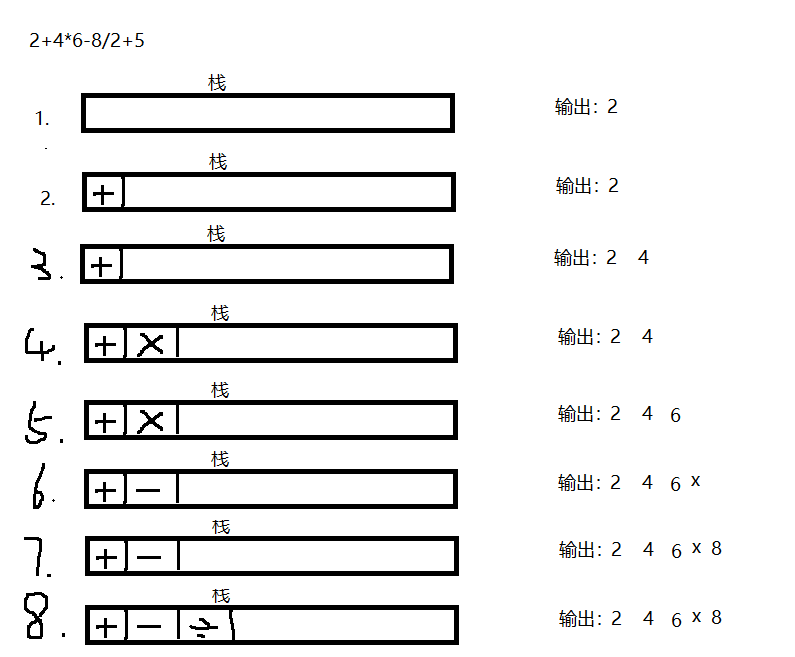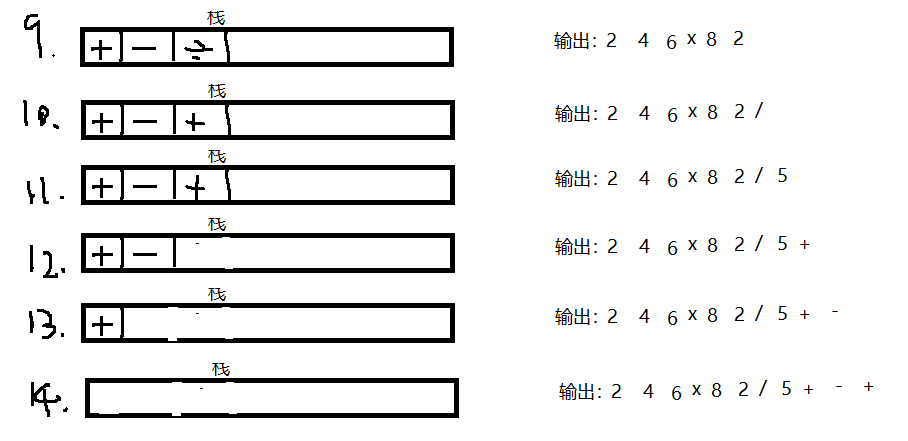四、代码实现：
#include <iostream>
#include <sstream>
using namespace std;

static int IDX = 0;			//压栈用
static int NUM_LEN = 0;		//查括号中的数字大小
static stringstream ss;

static string RESULT = ""; 	//结果缓存
/**
功能实现 :	中缀表达式转后缀表达式
@auther	 :
@date	 :
*/
class Stack{
private:
char data;			//存数字或者字符
public:
void setData(char data){
this->data = data;
}
char getData(){
return this->data;
}
Stack * next;		//指向下一个类
};

//遍历栈
void getStacks(Stack * top){
Stack * node;
node = top->next;
while(node != NULL){
RESULT += node->getData();
node = node->next;
}
}

//展示栈
void show(Stack * top){
Stack * node;
int i = 0;
node = top->next;
while(node != NULL){
cout<<node->getData();
node = node->next;
i++;
}
cout<<"\nthis stack length is :"<<i<<endl;
}

//删除指定符号
string delString(string str,int start,int end){
//然后通过erase进行删除：
str = str.erase(start,(end-start));           //从pos这个位置开始，删除1个字符
return str;
}

//最后结果展示
void resultView(){
string value = RESULT;
//判断结果是否存在[]号
int start =  RESULT.find('[');		//始
int end = RESULT.find(']');			//终
if(start == -1){
cout<<"result is :"<< value<<endl;
}else{
int cc = 0;
string len = RESULT.substr(start+1,(end-start-1));
ss << len;				//len = 4 ,cc = 44;
ss>>cc;
cc = cc % 10;			//
//插入括号
value.insert(start-cc,"(");
value.insert(end-1,")");
//删除[
start = value.find('[');
end = value.find(']');
value = delString(value,start,end+1);

cout<<"This result is: "<<value<<endl;
}
}

//查找改元素在栈内第几个
int select(Stack * top,char val){
Stack * node;
int i = 0;
node = top->next;
char resu;
while(node != NULL){
if(node->getData() == val){
return i;
}else{
node = node->next;
i++;
}
}
return -1;
}

//删除元素
char pop(Stack * top){
Stack * node;
node = top->next;
char val;
if(top->next == NULL){
return 0;
}else{
val = node->getData();
top->next = node->next;
delete(node);
return val;
}
}

//存入到栈里面
void push(Stack * top,char cc){
Stack * p = new Stack;
//判断  如果高级运算符再栈中就先放出再入栈
if(top->next == NULL){
p->setData(cc);
p->next = top->next;
top->next = p;
}else{
char one = (top->next)->getData();

if(cc == '('){			//遇到括号就压栈
IDX++;
p->setData(cc);
p->next = top->next;
top->next = p;
NUM_LEN = 0;		//开始计下标
} else if(cc == ')'){
//判断是否包含运算符
//存在运算符
int pero = select(top,'(');
if(pero > 0){	//存在运算符，不是纯数字
//出头栈并且不入栈再删除多一个栈
RESULT += pop(top);
pop(top); 		//出（号栈 ,不输出
IDX--;
}else{//不存在运算符，大于等于10的数
ss << NUM_LEN;
string s =  "["+ ss.str() +"]";
RESULT += s;
pop(top); 		//出（号栈 ,不输出
IDX--;
}

}

if(IDX == 0){
//one 为栈顶元素，如果进栈元素大于等于栈顶元素，就进栈，如果小于就输出栈顶元素
if(cc == '+' || cc == '-' ){
//cc 优先级小于等于栈头
//将头出栈，再新的运算符入栈
RESULT += pop(top);
p->setData(cc);
p->next = top->next;
top->next = p;

}else if(cc == '*' || cc == '/' ){
if(one == '+' || one == '-'){
//优先级大于栈顶，存入
p->setData(cc);
p->next = top->next;
top->next = p;
}else if(one == '*' || one == '/'){
//优先级等于，弹出
RESULT += pop(top);
p->setData(cc);
p->next = top->next;
top->next = p;
}
}
}else{
if(one == '('){
//任何字符直接存进去
p->setData(cc);
p->next = top->next;
top->next = p;
}else{	//	在括号里面已经存在有其他字符

if(cc == '+' || cc == '-' ){
//cc 优先级小于等于栈头
//将头出栈，再新的运算符入栈
RESULT += pop(top);
p->setData(cc);
p->next = top->next;
top->next = p;

}else if(cc == '*' || cc == '/' ){
if(one == '+' || one == '-'){
p->setData(cc);
p->next = top->next;
top->next = p;
}else if(one == '*' || one == '/'){
RESULT += pop(top);
p->setData(cc);
p->next = top->next;
top->next = p;
}
}
}
}
}
}

//输入一个中缀表达式
void input(Stack * top){
string str;
int idx = 0; 					//括号压栈
cout<<"cin:";
cin>>str;
for(int i=0;i<str.length();i++){
if(str[i] >= '0' && str[i] <= '9'){
//计算纯数字类型
if(IDX !=0 ){
NUM_LEN ++;
}
RESULT += str[i]; 		//遇到数字直接存进字符串
}else{
push(top,str[i]);		//这种+-先压栈，遇到*/直接弹出
}
}
}

int main(int argc, char** argv) {
//1.创建字符栈，存字符
Stack * top = new Stack;
top->next = NULL;
input(top);
//出完输入之后就从栈内找继续出
getStacks(top);
//展示最后的结果
resultView();
return 0;
}

五、运行结果：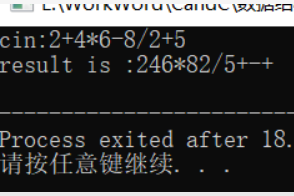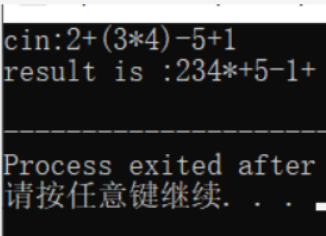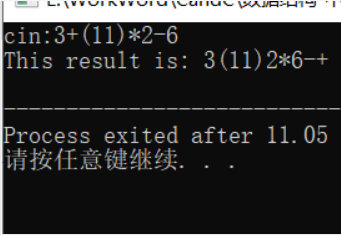六、备注：

1.1 两个括号以上的中缀表达式会出错，像2+((10)/2+1)+4这种，2+(10)-(11)+3;这种
1.2 不是整数的数进行运算也会报错
1.3 这只是写了个思路以及代码实现

转载请说明出处！！！

展开全文数据结构 c++ 中缀表达式 中缀转后缀
• } //中缀转后缀函数 bool reversePolish(ElemType str[],int length) { SqStack S;//声明符号栈 initStack(S);//符号栈初始化 string polish;//声明后缀表达式字符串 int i;//i是遍历中缀表达式的下标 for(i=0; i; ...
#include <iostream>
using namespace std;
#define MaxSize 50
typedef char ElemType;
typedef struct
{
ElemType data[MaxSize];
int top;
} SqStack;

//栈初始化
void initStack(SqStack &S)
{
S.top = -1;
}
//判断栈空
bool stackEmpty(SqStack S)
{
if(S.top == -1)
return true;
else
return false;
}
//入栈
bool push(SqStack &S,ElemType x)
{
if(S.top == MaxSize-1)
return false;
S.top++;
S.data[S.top] = x;
return true;
}
//出栈
bool pop(SqStack &S,ElemType &x)
{
if(S.top == -1)
return false;
x = S.data[S.top--];
return true;
}
//读栈顶元素
bool getTop(SqStack S,ElemType &x)
{
if(S.top == -1)
return false;
x = S.data[S.top];
return true;
}
//中缀转后缀函数
bool reversePolish(ElemType str[],int length)
{
SqStack S;//声明符号栈
initStack(S);//符号栈初始化
string polish;//声明后缀表达式字符串
int i;//i是遍历中缀表达式的下标
for(i=0; i<length; i++)
{
switch(str[i])
{
case '(':
push(S,str[i]);//左括号直接入栈
break;
case ')':
//遇到右括号，不入栈，弹出栈中（ 上面的所有符号并加入后缀表达式，最后把左括号弹出
ElemType topElem,x;
getTop(S,topElem);//读取栈顶元素
while(topElem != '(')
{
pop(S,x);
polish+=x;
if(stackEmpty(S))
{
//cout<<"栈空！"<<endl;
break;
}
else
getTop(S,topElem);
}

pop(S,x);
break;
case '+':
case '-':
//先弹出比自己级别高的或者与自己同级的运算符,加入后缀表达式
//cout<<"是+或者-，入栈"<<endl;
if(!stackEmpty(S))//如果栈非空
{
ElemType topElem;
getTop(S,topElem);//读取栈顶元素

while(topElem == '+' || topElem == '-' || topElem == '*' || topElem == '/')
{
ElemType x;
pop(S,x);
polish+=x;
if(stackEmpty(S))
{
//cout<<"栈空！"<<endl;
break;
}
else
getTop(S,topElem);
}
}
push(S,str[i]);//弹出后自己入栈
break;
case '*':
case '/':
//先弹出比自己级别高的或者与自己同级的运算符,加入后缀表达式
//cout<<"是*或者/，入栈"<<endl;
if(!stackEmpty(S))//如果栈非空
{
ElemType topElem;
getTop(S,topElem);//读取栈顶元素

while(topElem == '*' || topElem == '/')
{
ElemType x;
pop(S,x);
polish+=x;
if(stackEmpty(S))
{
// cout<<"栈空！"<<endl;
break;
}
else
getTop(S,topElem);
}
}
push(S,str[i]);//弹出后自己入栈
break;
default:
polish+=str[i];
}
}
while(!stackEmpty(S))
{
ElemType x;
pop(S,x);
polish+=x;
}
cout<<"后缀表达式为："<<endl;
cout<<polish;
return true;
}
int main()
{
ElemType str[MaxSize];
int length;
cout<<"请输入中缀字符串长度：";
cin>>length;
cout<<"请输入中缀表达式："<<endl;
cin>>str;
cout<<"您输入的中缀表达式为：";
cout<<str<<endl;
reversePolish(str,length);
return 0;
}

代码有个缺点，输入的时候必须输入要测试的中缀字符串长度，我懒得改了~~其他应该还是没问题的

展开全文c++ 字符串 数据结构 正则表达式
• 主要为大家详细介绍了C++实现中缀表达式转后缀表达式，文中示例代码介绍的非常详细，具有一定的参考价值，感兴趣的小伙伴们可以参考一下
• 用dev c++写的代码，附有啰里啰嗦的注释和测试样例。太简单了不好意思要分。
• 用栈实现表达式中缀转后缀 栈的特点是先进后出，可以用来将中缀表达式转换成后缀表达式，在本程序中对表达式进行了限制，数字0-9，运算符只有±*/（），缩小了问题规模。 转换思路：将表达式输入，全部数据以char型...

用栈实现表达式中缀转后缀

栈的特点是先进后出，可以用来将中缀表达式转换成后缀表达式，在本程序中对表达式进行了限制，数字0-9，运算符只有±*/（），缩小了问题规模。

转换思路：将表达式输入，全部数据以char型存储在vector容器中，输入完后从左到右扫描vector，遇到数字直接输出，遇到操作符时根据操作符的种类分为三种情况进行操作：

1.遇到*/和( 时直接入栈，乘除运算级别最高，所以应该直接入栈，左括号也直接入栈，直到遇到右括号才出栈。

2.遇到±时，若栈中无元素，则直接入栈，否则开始出栈直到遇到左括号或者栈，再入栈。

3.遇到右括号开始出栈，知道遇到 左括号，其中左括号不输出。

结点的定义

typedef struct //顺序栈的结构定义
{
char *base;
char *top;
int stacksize;
}SqStack;

代码实现如下
下面展示一些 内联代码片

#include <iostream>
#include<vector>
using namespace std;

#define MAXSIZE 100

typedef struct //顺序栈的结构定义
{
char *base;
char *top;
int stacksize;
}SqStack;

void InitStack(SqStack &S)//初始化
{
S.base = new char;
if (!S.base)
{

cout << "内存空间不足" << endl;
exit(1); //空间不足 退出
}

S.top = S.base;
S.stacksize = MAXSIZE;
}

void Push(SqStack &S, char e) //入栈
{
if (S.top - S.base == S.stacksize)
{
cout << "栈已满" << endl;
exit(1);
}

*S.top = e;
*S.top++;

}

void Pop(SqStack &S, char &e) //出栈并返回栈顶元素
{
if (S.top == S.base )
{
cout << "栈已空" << endl;
exit(1);
}

*S.top--;
e = *S.top;
}

int main()
{
char e;    //e用来暂时存出栈的元素
SqStack Result;
vector<char> data; //储存表达式的容器
char mydata;

void InitStack(SqStack &S); //初始化栈
void Push(SqStack &S, char e); //压栈
void Pop(SqStack &S,char &e); //出栈

InitStack(Result);
cout << "输入中缀表达式，以CTRL + Z结束" << endl;

while (cin >> mydata)  //输入表达式，以CTRL+Z结束。
data.push_back(mydata); //vector容器，在尾端添加元素的方法

//下面开始从左到右依次扫描data数组；

for (int i = 0; i < data.size(); i++ )   //从左到右开始扫描整个中缀表达式
{

if (data[i] >= '0' && data[i] <= '9') //数字直接输出
cout << data[i] << " ";

else if(data[i]=='*'||data[i]=='/'||data[i]=='(') //最简单的情况 */（ 直接入栈
{
Push(Result, data[i]);
}

else if(data[i] == '+' || data[i] == '-')
{
if( Result.base == Result.top )//如果栈空，则直接将+-压入栈里
{
Push(Result, data[i]);
}

else
{

do
{
Pop(Result, e);
if(e == '(')
{
Push(Result,e);
}
else
{
cout << e;
}
}while( Result.base != Result.top && e != '(' );

Push(Result,data[i]);
}

}

else if ( data[i] == ')')
{
Pop(Result,e);

while(e!='(')
{
cout << e << " ";
Pop(Result,e);
}
}
else cout <<"ERROR";

}
while(Result.base != Result.top)
{
Pop(Result, e);
cout <<e << " ";
}

return 0;

}

展开全文数据结构 c++
• 中缀转后缀表达式计算实现源码（C++、Java）
• 主要为大家详细介绍了C++利用栈实现中缀表达式转后缀表达式，文中示例代码介绍的非常详细，具有一定的参考价值，感兴趣的小伙伴们可以参考一下
• 将用户在终端输入的中缀表达式转换成后缀表达式，并对输入格式进行检查
• 　中缀表达式就是我们通常所书写的数学表达式，后缀表达式也称为逆波兰表达式，在编译程序对我们书写的程序中的表达式进行语法检查时，往往就可以通过逆波兰表达式进行。我们所要设计并实现的程序就是将中缀表示的...中缀转后缀
• C++中缀表达式转后缀表达式

千次阅读 多人点赞 2019-01-03 23:44:47
一、思路：和中缀表达式的计算类似，只不过不用计算，把表达式输出即可  1.用字符数组存储整行输入的中缀表达式；  2.接着从字符数组的0位置开始判断字符，如果是数字，那就要判断后面是否是数字，如果是就不断...

一、思路：和中缀表达式的计算类似，只不过不用计算，把表达式输出即可

1.用字符数组存储整行输入的中缀表达式；

2.接着从字符数组的0位置开始判断字符，如果是数字，那就要判断后面是否是数字，如果是就不断扫描组成一个整数

（暂不考虑负数和小数），最终组成一个整数，然后输出这个数（因为不用计算，所以直接输出即可）；

3.如果是左括号，直接进符号栈；

4.如果是操作运算符，与符号栈的栈顶元素比较优先级：如果高就压入栈；

低，就取出符号栈顶的元素输出；

接着，再判断符号栈顶的元素和当前的运算符号继续比较优先级，重复前面步骤，直到栈空或者当前的符号优先级高；

5.如果是右括号，把符号栈栈顶的元素取出，如果不是左括号，把取出的运算符输出，接着取符号栈栈顶的元素，直到符号栈

中取出的符号是左括号；

6.当扫描完字符数组时，判断符号栈是否为空：

不为空，把符号栈栈顶的元素取出，输出到窗口，直到符号栈为空。

二、实现程序：

//  中缀表达式转后缀表达式
//  操作符：+、-、*、／、%
//  输入：可以用cin.getline(arr, 250)或者cin.get(ch) && ch != '\n'
//  测试数据：输入格式：(注意：不能有中文的操作符)
//           2+(3+4)*5
//           16+2*30/4
//      输出格式：
//          2 3 4 + 5 * +
//          16 2 30 * 4 ／ +

#include <iostream>
#include <stack>

// 判断是否是操作符
bool isOperator(char ch) {
if(ch == '+' || ch == '-' || ch == '*' || ch == '/')
return true;
return false; // 否则返回false
}

// 获取优先级
int getPriority(char ch) {
int level = 0; // 优先级

switch(ch) {
case '(':
level = 1;
break;
case '+':
case '-':
level = 2;
break;
case '*':
case '/':
level = 3;
break;
default:
break;
}
return level;
}

int main(int argc, const char * argv[]) {
// insert code here...
int num;
char arr; // 一个一个的读取表达式，直到遇到'\0'
std::stack<char> op; // 栈op:存储操作符

while(1) {
std::cin.getline(arr,250);
int len, i;
char c; // c存储从栈中取出的操作符

len = (int)strlen(arr); // strlen()输出的是：unsigned long类型，所以要强制转换为int类型
i = 0;
while(i < len) {
if(isdigit(arr[i])) { // 如果是数字
num = 0;
do {
num = num * 10 + (arr[i] - '0'); // ch - 48根据ASCAII码，字符与数字之间的转换关系
i++; // 下一个字符
}while(isdigit(arr[i]));
std::cout << num << " ";
} else if(arr[i] == '(') { // (:左括号
op.push(arr[i]);
i++;
} else if(isOperator(arr[i])) { // 操作符
if(op.empty()) {// 如果栈空，直接压入栈
op.push(arr[i]);
i++;
}
else {
// 比较栈op顶的操作符与ch的优先级
// 如果ch的优先级高，则直接压入栈
// 否则，推出栈中的操作符，直到操作符小于ch的优先级，或者遇到（，或者栈已空
while(!op.empty()) {
c = op.top();
if(getPriority(arr[i]) <= getPriority(c)) {
// 优先级低或等于
std::cout << c << " ";
op.pop();
} else // ch优先级高于栈中操作符
break;
} // while结束
op.push(arr[i]); // 防止不断的推出操作符，最后空栈了;或者ch优先级高了
i++;
} // else
} else if(arr[i] == ')') { // 如果是右括号，一直推出栈中操作符，直到遇到左括号(
while(op.top() != '(') {
std::cout << op.top() << " ";
op.pop();
}
op.pop(); // 把左括号(推出栈
i++;
} else // 如果是空白符，就进行下一个字符的处理
i++;
} // 第二个while结束
while(!op.empty()) { // 当栈不空，继续输出操作符
std::cout << op.top() << " ";
op.pop();
}
std::cout << std::endl;
flush(std::cout);
} // 第一个while结束
return 0;
}

运行结果：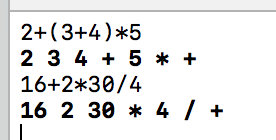展开全文C++ 中缀表达式 后缀表达式
• C++基于栈的建议计算器，支持+、-、*、/ 的基本运算。输入中缀表达式，转化成后缀表达式后再输出计算结果。自动检查括号不匹配、除数为0等异常。
• 一、如何将中缀表达式转化为后缀表达式 在日常应用中，算术表达式中运算符总是出现在两个操作数之间，例如5*(7-23)+8/2，这种形式称为中缀表达式。计算一个中缀表达式需要知道运算符的优先级和结合...
• 中缀转后缀c++实现

千次阅读 2013-08-26 16:50:40
表达式的表示形式有中缀、前缀和后缀3中形式。中缀表达式按操作符的优先级进行计算（后面代码实现只包括+、-、*、\,小括号），即数学运算。后缀表达式中只有操作数和操作符。操作符在两个操作数之后。它的计算规则...
• 一个算术表达式是由操作数(operand)、运算符(operator)和括号组成的。...用户可选择需要进行的操纵，包括后缀表达式的计算，中缀表达式转为后缀表达式，清屏和退出，界面清晰，操作简便，且有足够的输入合法性检验
• } } string transfer(string str) {//中缀转后缀 stack<char>S; int rank; string ans = "";//ans为最终答案，以字符串形式存储 int i = 0; while (str[i]!='\0') { if (i > 0 && ('0' > str[i - 1] || str[i...c++
• 数据结构课程实验，实现了终端输入输出的中缀表达式求值，后缀表达式求值，中缀表达式转化后缀表达式，以及文件流的中缀表达式求值，后缀表达式求值，中缀表达式转化后缀表达式，可操作整数，小数，浮点数，实现运算...
• calc_res里面使用空格分割是个很不好的情况..需要保证转换的后缀式+-/*...//数据结构与算法分析 栈c++实现 //缺点 需要一次性分配足够的栈空间 没有实现动态变长 //优点 大部分操作的消耗都是常量级别的 template
• C++写的一个中缀转后缀表达式并计算结果的一个程序！C++ 中缀转后缀
• 算法：中缀表达式转后缀表达式（想看全部代码直接翻到底部） 欢迎大家进入二阳的学习课堂！！希望大家耐心看下去，这样才能有收获。（想看全部代码直接翻到底部） 众所周知，在编程里面，直接用中缀表达式求值是不...算法 计算表达式
• c语言实现中缀表达式转后缀表达式并求得计算结果，用顺序栈结构。 当输入者输入错误信息的时候需要报错，并说明错误的种类。
• 我们分析下，后缀表达式的运算法则非常简单 1.从前往后扫描，数字不做处理。 2.遇到运算符，则取运算符前两个数字进行计算。 3.用计算结果替代原来两个数字和运算符，并从新从第一步开始运行，直到只有一个数字为止...数据结构 算法 c++
•数据结构 c++
• 中缀表达式，也就是我们常见常用的表达式一般形式： 比如 6+7*(2+3)+10/5 而后缀表达式，则是在中缀表达式基础上，进行一定规则的转换： 1、按运算符优先级对所有运算符和它的运算数加括号，（原本的括号不用加） 2...c++ 字符串 数据结构
• 很好的哦~ 表达式的计算（中缀转后缀再计算） 表达式的计算（中缀转后缀再计算）...

中缀转后缀c++c++ 订阅